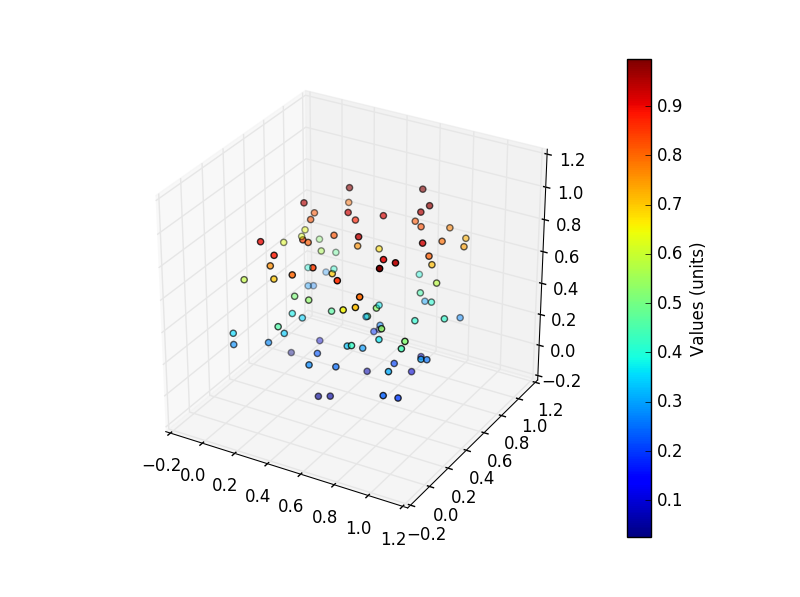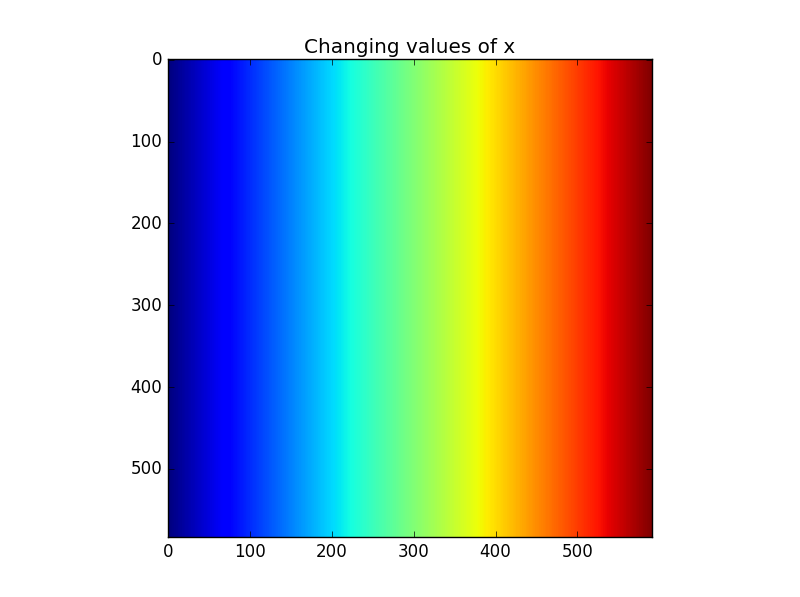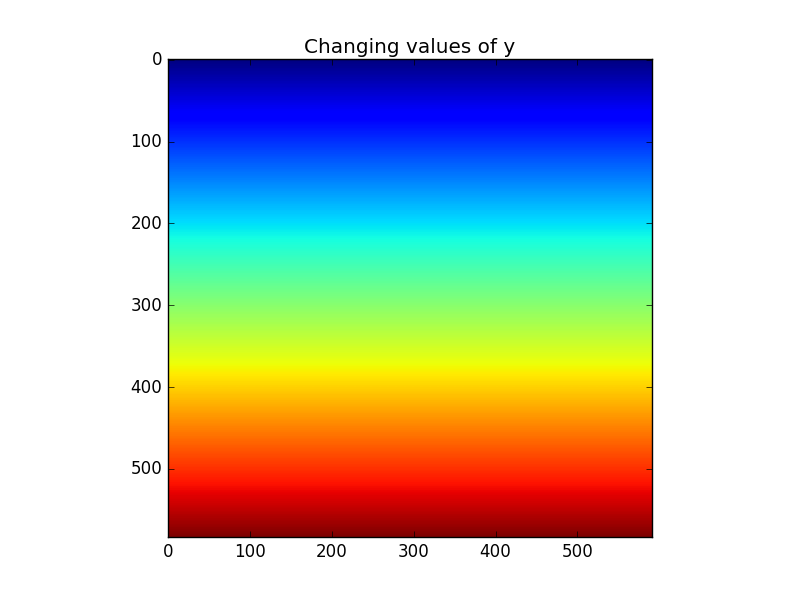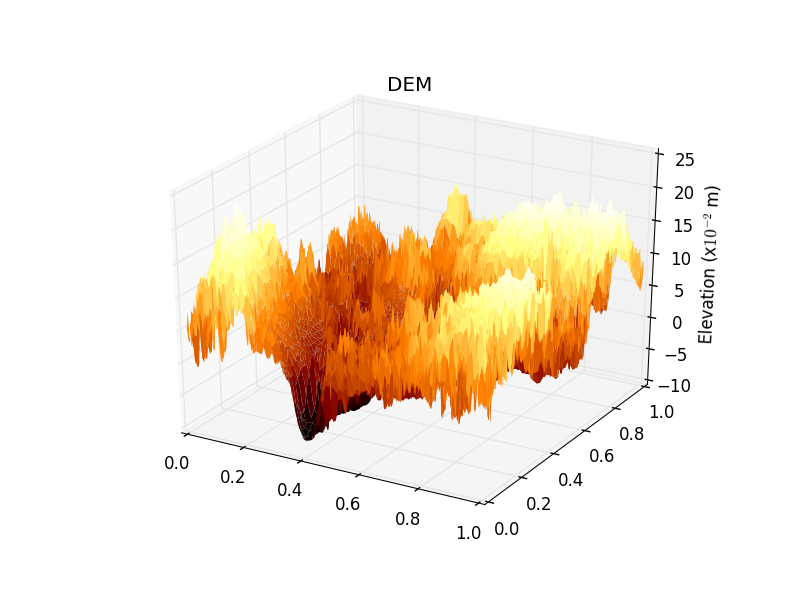# 3D plotting with matplotlib

There are a number of options available for creating 3D like plots with matplotlib. Let’s get started by first creating a 3d scatter plot.

## 3D scatter plot

Let’s first create some data:

``````import numpy as np
xyz=np.array(np.random.random((100,3)))
``````

and assign it to specific variables (for clarity and also to modify the z values):

``````x=xyz[:,0]
y=xyz[:,1]
z=xyz[:,2]*100
``````

Now we need to import the 3d package:

``````import matplotlib.pyplot as plt
from mpl_toolkits.mplot3d import Axes3D
``````

To create our 3D plot, we must take a slightly different approach which will provide us with greater opportunity for plot customisation. First we will create and assign a figure object:

``````fig = plt.figure()
``````

Now, from the figure object we are going to create a subplot (of which there will only be one) - the need to do this is to ensure that we have specific access to the properties of the figure we are creating (before, where we called say `plt.scatter()` we were unable to specifically access its axis properties other than by default):

``````ax = fig.add_subplot(111, projection='3d')
``````

We will revisit what is meant by the `111` later on in the multiple plots section. For now, have a look at the number of options now available to you for modifying the axis object by typing `ax.` followed by the tab key.

The 3D scatter plotting function (`Axes3D.scatter()`) takes in x, y and z values which we can set using our `xyz` array object:

``````ax.scatter(x,y,z)
``````

which we can then show (or even save) as normal - have a go at interacting with the figure that pops up:

``````plt.show()
``````

To add a colorbar, we need to assign the definition of the scatter plot to a variable which we then pass to the colorbar function. First, re-assign the figure and axis variables:

``````fig = plt.figure()
``````

Now recreate the scatter plot and assign it ot a variable name (we call ours pnt3d) - to make the colorbar work, you must also set the `c` option - as we are using our `z` variable to colour our pioints, we set this as `c=z`:

``````pnt3d=ax.scatter(x,y,z,c=z)
``````

Now create your colorbar, and pass in the scatter plot (called `pnt3d`):

``````cbar=plt.colorbar(pnt3d)
``````

Using the colorbar object (`cbar`), we can also give it a title:

``````cbar.set_label("Values (units)")
``````

We can then plot this as normal:

``````plt.show()
``````

and you should end up with something like this:## 3D surface plot

To make a 3D surface plot, we can reuse the dem we opened before (which you can save using this link).

Read this in as a numpy array using `scipy.misc.imread`:

``````from scipy import misc
``````

The function to plot 3d surfaces is available as for the 3d scatter plot demonstrated above - it can be imported as follows:

``````import matplotlib.pyplot as plt
from mpl_toolkits.mplot3d import Axes3D
``````

Notice that we have set an alias for each of the imports - `plt` for `matplotlib.pyplot` and `Axes3D` for `mpl_toolkits.mplot3d`.

If you have a look at the documentation for mpl_toolkits.mplot3d.plot_surface (which you can access using your alias by typing `Axes3D.plot_surface?`), you will see that it takes in x, y and z values that must all be 2D arrays - the problem at the moment is that your surface array (`dem`) only provides the z data - you don’t have the x or y components.

To create this, we can use a function from numpy called meshgrid. For a given array of values, the meshgrid function creates 2 equally sized grids that represent the x and y location at each element of the array, that is to say that for an element in our `dem` array, the x and y mesh grids will provide information of its location in x and y space… let’s try an example…

First, check on the shape of your `dem` array:

``````>>> dem.shape
>>> (592L, 584L)
``````

Now import `numpy` - we’ll give it an alias of `np`):

``````import numpy as np
``````

Now we need to create the dimensions of what will be our mesh grids of x and y. First assign the dimensions of the `dem` array to variables of `nx` and `ny`:

``````ny, nx = dem.shape
``````

The above statement assigns the two values returned by the `dem.shape` call to the two variables `nx` and `ny`.

We now need to make two lists of values ranging from 0 to the maximum length of the image in both the x and y dimensions - we can do this by using numpy’s linspace function:

``````x = np.linspace(0, 1, nx)
y = np.linspace(0, 1, ny)
``````

This has created every likely element position in the x and y dimensions - remember that for a given column, the x value will remain the same (now matter how far up or down the column it is) and the same for y in the horizontal direction - if this doesn’t make sense, we’ll have an image to look at in a minute.

Now we just need to pass in the `x` and `y` variables to `np.meshgrid()` to get our arrays of x and y position (calling the resultant grids `xv` and `yv`):

``````xv, yv = np.meshgrid(x, y)
``````

To visualise these grids, here is x, the values of which only change from left to right:and here is y, the values of which only change from top to bottom:The key point now is that we have 3 2d arrays, representing x, y and z, held by the variables `xv`, `yv` and `dem` respectively. Now we can pass these into the `Axes3D.surface_plot()`. We have to do this in the same way as for the 3d scatter plot above, so type:

``````fig = plt.figure()
dem3d=ax.plot_surface(xv,yv,dem)
plt.show()
``````

To adjust the colours, set the type of colormap you want to use using the `cmap` option when creating the main plot:

``````dem3d=ax.plot_surface(xv,yv,dem,cmap='afmhot')
``````

You might also want to add a title and axis labels to the plot - as we are using a specific call to the plot axis, we must set this using:

``````ax.set_title('DEM')
ax.set_zlabel('Elevation (m)')
``````

You can then view it using:

``````plt.show()
``````

If you prefer a smoother looking image, then you want to adjust the `linewidth` option when creating the plot:

``````dem3d=ax.plot_surface(xv,yv,dem,cmap='afmhot', linewidth=0)
``````

You might also like to play with the `alpha` option which changes transparency:

``````dem3d=ax.plot_surface(xv,yv,dem,cmap='afmhot', linewidth=0, alpha=0.2)
``````

If you want to change the scale of the values in the `dem` array, then you are best modifying the values before plotting it e.g.:

``````dem_100=dem/100
``````

Remember that if you make these changes, you need to then recreate the figure and axis instances. So, after making some additional changes, we create the figure by typing:

``````fig = plt.figure()